Journal of Electrical and Electronic Engineering
Volume 4, Issue 2, April 2016, Pages: 18-23

Comprehensive Analysis on Characteristics of SiC Power Device

Shi Mingming1, Lu Wenwei2, Ge Le2

1Jiangsu Electric Power Research Institute, Nanjing, China

2Nanjing Institute of Technology, Nanjing, China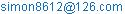(Shi Mingming)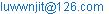(Lu Wenwei)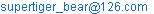(Ge Le)

Shi Mingming, Lu Wenwei, Ge Le. Comprehensive Analysis on Characteristics of SiC Power Device. Journal of Electrical and Electronic Engineering. Vol. 4, No. 2, 2016, pp. 18-23. doi: 10.11648/j.jeee.20160402.13

Received: March 17, 2016; Accepted: March 31, 2016; Published: April 7, 2016

Abstract: Analyzing the research status and development trend of SiC (Silicon Carbide) power device, this article describes the latest research results of switching characteristics and power loss characteristics of SiC power device. With detailed analysis on switching characteristics of Schottky Barrier Diode (SBD) and MOSFET, this paper emphasizes on the differences between them and the corresponding power devices. The comparison study between power loss characteristics of MPS and valve loss of silicon carbide thyristor for ultra-high voltage, also and the differences in power loss of switching power supply between SiC MOSFET and Si MOSFET provide scientific basis for the optimal selection and application of SiC power device.

Keywords: SiC, Power Device, Switching Characteristics, Power Loss Characteristics

1. Introduction

Power electronic devices are the vital basis of power electronic devices. The characteristics of the devices have vital effects on the technical index and performance of the device . Nowadays, the outstanding advantages of SiC materials encourage researchers to research and develop SiC power electronic devices with high performance, actively promoting its commercialization process to earn a wider range of application advantage and potential. In the field of high frequency, high temperature and high power electronic applications, advantages and great application of power electronic devices SiC power electronic devices has the incomparable potential to Si semiconductor device .

SiC power devices commercialization greatly promoted the study of applied technology SiC power devices, particularly in the areas of aerospace, electric vehicles, exploration, power systems and new energy power generation, researchers system-level benefits assessment of the SiC power device, drive circuit design, multi-tube technology and the expansion of power converters to achieve high temperature and many other technical problems explored and studied.

Currently, SiC SBD, SiC JFET and SiC MOSFET have successfully commercialized. Due to the different characterisctics of semiconductor materials, there are some differences in the electrical characteristics between SiC power devices and Si power devices [2-6]. In order to ensure the correct use of SiC power devices and give full play to its advantages, which based on the converter system of SiC power devices can obtain better performance, we need thorough analysis and study on switching characteristics, loss characteristics and parameters of SiC power device, especially on switching characteristics and loss characteristics [5-7].

With rapid development of silicon carbide materials, SiC power semiconductor devices have been widely concerned in the field of switching power supply, being a potential alternative to the Si semiconductor devices. In order for better application of SiC power devices, this paper studies the switching characteristics of SiC SBD and MOSFET, the power loss characteristics of MPS, valve loss of Silicon Carbide thyristor for ultra-high voltage and the differences in power loss of switching power supply between SiC MOSFET and Si MOSFET.

2. Analysis on Switching Characteristics of SiC SBD

Switching characteristics of power diode includes forward recovery characteristics and reverse recovery characteristics. The following analysis is respectively on SiC and SBD .

2.1. Forward Recovery Characteristics

The formation of power diode voltage overshoot is mainly related to two factors: conductivity modulation effect and internal parasitic inductance effect. Due to the absence of conductivity modulation effect, SBD SiC can only be affected by parasitic inductance, and the zero forward recovery voltage of SBD SiC can be basically achieved by process improvement.

2.2. Reverse Recovery Characteristics

Reverse recovery characteristics of power diode is an important index of diode selection, mainly affected by the conductivity modulation effect and parasitic capacitance effect. Si fast recovery diode has conductivity modulation effect, with long reverse recovery time and large spike reverse recovery current. It can induce large voltage spikes on the stray inductance in the line, which may increase the voltage stress of power device. SBD SiC has no conductivity modulation effect, and reverse recovery is mainly affected by the parasitic capacitance in the circuit. As a result, the reverse current spike is small, the switching speed is fast, and the switching loss is small too.

3. Analysis on Switching Characteristics of SiC MOSEFT

3.1. Switching Characteristics

The switching characteristics of SiC MOSFET are mainly concerned with the nonlinear parasitic capacitance of poles. Table 1 gives the similar power level (1200V/10A) of SiC MOSFET and input capacitance Ciss, output capacitance Coss and Miller capacitance Crss capacitance value of Si MOSFET [9, 10]. These parasitic capacitance parameters have obvious influence on the transient switching process of power MOSFET.

Table 1. Comparison of MOSFET Parasitic Capacitance.

 Devices Types Ciss(pF) Coss(pF) Crss(pF) CMF10120D(SiC) 928 63 7.45 IXTH12N120(Si) 3400 280 105

From table 1, the parasitic capacitance values of all poles of MOSFET SiC are much less than that of Si MOSFET in the similar power levels. Based on the switching process of MOSFET, the smaller parasitic capacitance value is, the faster switching speed of MOSFET can be, which can shorten the switching process time. So as to reduce the transition cross region of leakage current and drain source voltage in the switching process, that is to say, it can reduce the switching loss of the MOSFET.

3.2. Driving Characteristics

The performance of the grid drive circuit plays a key role of on the switching process of MOSFET. In the fast switching application of SiC MOSFET, we especially need to seriously consider the existing problems in the driving circuit .

From the point of reducing the conduction resistance, it is beneficial to set the driving voltage of SiC MOSFET higher. But for the fast switching process of SiC MOSFET, the current rate of change of drain is large and the voltage rate of change of drain source is large, through the parasitic inductance and the miller capacitance in the circuit, it coupled to the grid and connected to the source electrode through the grid resistor, forming a loop eventually. Both ends of grid resistance will cause serious voltage spike. Due to the possible breakdown phenomenon for the grid in the switching process of SiC MOSFET, the value of positive driving voltage should be limited.

In the shutdown process, due to the lower open voltage of SiC MOSFET, the grid voltage spike may lead to mis-conducting. So we need to set up the negative bias voltage to enhance the anti interference ability of the grid. The most direct way to control the coupling is to reduce the parasitic parameter in the circuit and to reasonably select the driving resistance of external grid.

4. Analysis on Power Loss Characteristics of Silicon Carbide MPS

MPS diode is an integrated diode device on forming a net PN junction in the drift region of Schottky diode. The net structure design as shown in Figure 1 makes the depletion region of PN junction not be interlinked in the positive and zero situation. When it works positively in this way, with current flowing through multiple conductive channels under the Schottky barrier, the device is conductive. When it works negatively, PN junction and Schottky barrier is reverse biased, making the depletion region formed by a PN junction extend to the channel region. As soon as the reverse bias voltage is more than a certain value, the depletion layer of the Schottky barrier will overlap .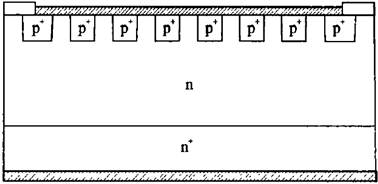Figure 1. The structure of MPS diode.

When the depletion layer passes through, it will form a potential barrier in the channel region. So as to increase the reverse bias voltage from the depletion layer to the direction of the N+ substrate, it can support this barrier and shield the Schottky barrier from the voltage bias, suppressing the Schottky barrier reduction effect and eliminating the leakage current. As soon as the penetrating condition establishes, the other parameters stay constant in addition to the slight increase in the external leakage current caused by the space charge region.

Power consumption of device is determined by the I-V characteristics. For 4 H-SiC MPS devices, power consumption is composed of three parts: the first one is power consumption in forward conduction, decided by forward voltage dropping, forward conduction current and working cycle; the second one is power consumption in reverse blocking, depending on the reverse bias voltage, leakage current and working cycle; the third one is power consumption in switching process, due to the fast switching speed of MPS diode, the static power consumption is the main part.

4.1. Power Consumption in Forward Conduction

Assuming the forward conduction current is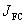, the conduction time is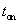, the total working time is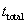. The power consumption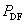can be calculated by the following formula: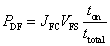(1)

where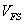is forward voltage drop of Schottky barrier and drift region.

4.2. Power Consumption in Reverse Blocking

Assuming the imposed bias voltage when reverse blocking is VR, the conduction time is, the total working time is. The power consumption in reverse blocking PDR is concluded from the following formula: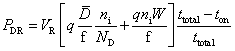(2)

Combining the power consumption in forward conduction and reverse blocking of MPS, we can conclude the total power consumption PD: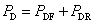(3)

4.3. How Temperature Effect Power Consumption

When the device temperature rises, height of Schottky barrier, ionization rate of drift region impurity, electron mobility, diffusion coefficient, life and the intrinsic carrier concentration and so on, these factors will change, which also lead to the power consumption of MPS. Because SiC has a "frozen" effect that is not completely ionized at room temperature, and the ionization rate changes with the change of temperature, we must take how temperature effect power consumption into consideration when calculating the relationship between temperature and power consumption.

In the temperature range of 300900K, the intrinsic carrier of material SiC changes intensely with temperature. Although the diffusion coefficient and the lifetime of the electrons change with temperature, the change of the intrinsic carrier concentration is the main factor affecting the power consumption during reverse blocking.

5. Analysis of Valve Loss Characteristic of Ultra-High Voltage DC Silicon Carbide Thyristor

5.1. Circuit Model

Ultra-high voltage DC transmission system based on thyristor valve is a complex system composed of many electrical components. The situation is more complicated if the exchange system is considered for the coupling of the converter transformer. The circuit model is simplified without sacrificing the equivalence principle in this paper. We use ideal voltage source for AC system. Taking the need of commutation circuit into account, the converter transformer adopts the ideal transformer model with series transformer leakage inductance and think of existing stray capacitance of transformer lead wire end. Single pole converter circuit model is shown in Figure 2 [13-16].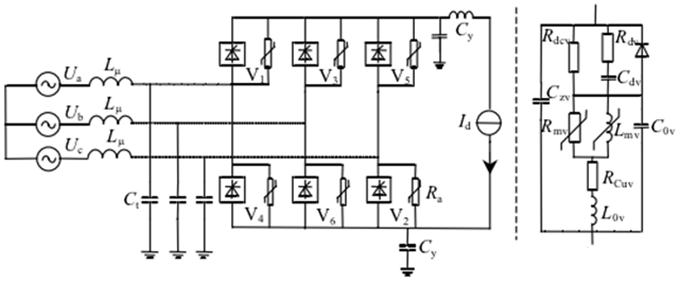Figure 2. Working Circuit of Converter Valve.

Lμ—leakage inductance of converter transformer; Ct—stray capacitance from AC side; CZV—valve terminal capacitance; Rdcv—valve equivalent DC equalizing resistor; Rdv—valve damping resistance; Cdv—valve damping capacity; Rmv—equivalent resistance of valve reactor; LmV—equivalent inductance of valve reactor; RCuV—DC coil resistance of valve reactor; L0V—stray inductance; C0v—equivalent capacitance of valve reactor; Cy—stray capacitance from DC side; Ra—valve arrester.

5.2. Electrical Design Parameters of Converter Valve

The DC converter valve thyristor contains two design schemes in this paper: 1) use conventional commercial silicon devices, that is, Zhuzhou KPE5000-72 thyristor; 2) Silicon carbide KPD5000-400 thyristor is calculated by the characteristics of silicon carbide material, and the main parameters are compared as shown in Table 2 .

Table 2. Comparison of Main Parameters of Silicon Thyristor and Silicon Carbide Thyristor.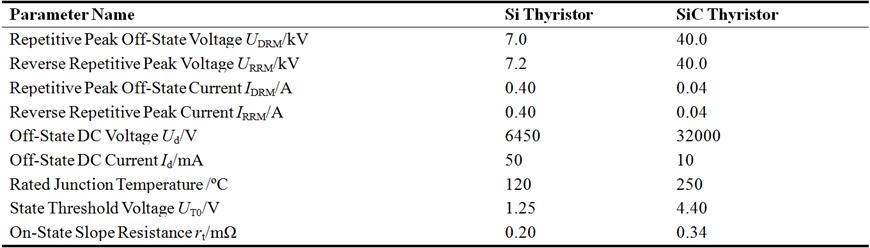Due to the increase of the voltage resistance of silicon carbide single thyristor, a large number of thyristor series are reduced. The calculation of each single valve (pressure 200 kV) SIC thyristor series number is 12, but the silicon thyristor series number is 67.

When the converter valve is close to 90 degree, and when the load current is the maximum value of the silicon flow valve and silicon carbide flow valve, the operating parameters are shown in table 3. In the use of silicon carbide thyristor valve, when the valve is close to 90 degrees, we assume that the impact of the saturation reactor is unchanged. As the valve current passes zero, di/dt is constant. According to the reverse recovery charge relationship between silicon carbide thyristor and silicon thyristor, combining the reverse recovery charge characteristic curve, we can calculate the reverse recovery current peak value IRM=130A and the reverse recovery charge is Qn=760μC.

Table 3. Circuit Parameters of Silicon Valve and Silicon Carbide Valve.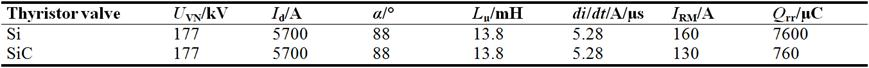5.3. Method for Calculating Loss of Converter Valve Unit

The circuit theory points out that the instantaneous power consumption of a circuit element is equal to the product of voltage and current on both ends of the element (P=UI), and if the circuit element is a resistance element, the power consumption of the resistance element is converted into Joule heat. In a working cycle T, the average loss of the resistive element is: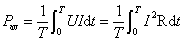(4)

In the study of the basic components of commutation valve's electrical model, the electrical model of the thyristor under different working conditions is a represent of series of resistance, voltage or voltage source and the resistor; Rm represents core loss of saturable reactor, RCu represents saturated reactor winding. The loss of each component can be calculated in accordance with the formula (4) as long as the voltage and current at the ends of these resistors are determined.

5.4. Loss of Converter Valve and Junction Temperature of Thyristor Under Different Working Conditions

Calculated by the loss of the converter valve components, the commutation components will inevitably generate losses at runtime. Loss associated with thyristor (loss of state, loss of opening, loss of turn off and loss of off state) will increase the junction temperature of thyristor. The blocking ability of thyristor is closely related to the junction temperature of thyristor. If the thyristor junction temperature exceeds the rated junction temperature, the thyristor may lose its ability to block, resulting in the loss of the normal operation of the converter valve . In order to ensure the junction temperature of thyristor, the exsiting DC converter valve is equipped with water cooling system.

5.5. Economic Benefits of Silicon Carbide Thyristor

It can be seen that the SiC thyristor can reduce the loss of the DC converter valve under various working conditions. These savings can be provided to the user, bringing the dual benefits of energy saving, environmental protection and economic profit. Taking ±800 kV, 5 kA DC exchange commutation as an example, we estimate the direct economic benefits to use silicon carbide single valve instead of silicon single valve. Assume the DC converter valve is under the rated operating conditions in 365 days of each year . Silicon carbide single valve power loss is 136kW, so the use of silicon carbide single valve annual power consumption is 397MW, and the use of a single valve annual power consumption is 726MW. In a DC project, if the use of SiC thyristor valve can save 397MW·h annually, according to the ordinary residents electricity fee 0.5 yuan/kW·h to calculate, the electricity loss annual saved can directly gain profit of 17.5 million yuan.

6. Comparative Analysis of Power Loss of SiC MOSFET and Si MOSFET in Switching Power Supply

6.1. Power Loss Analysis of Switching Power Supply

The power loss of MOSFET in switching power supply is mainly the conduction loss and switching loss . Specific analysis are shown as follows:

6.1.1. Conduction Loss

Conduction loss of MOSFET PQ in switching power supply is decided by the conduction resistance RQ(on), the calculation of conduction loss PQ is shown in the formula (5):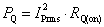(5)

where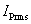is the current value flowing through the switching tube. Under the condition of keepingthe same, the PQ is proportional to the conduction resistance RQ(on). The size of RQ(on) is changing with the junction temperature T of MOSFET, Specific relationship is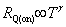. where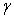is a constant,of Si is 2.42, butof SiC is 1.3 . In the high junction temperature of 135 C, the resistance of SiC MOSFET is only increased by 20% while the Si MOSFET is up 240%. Therefore, the SiC MOSFET device is suitable for working under high temperature environment, and comparing with Si MOSFET device, only smaller heat sink is required.

6.1.2. Switching Loss

Switching losses are generated due to the switching time of the MOSFET. In the process of MOSFET's communication and interruption, due to the effective voltage and current working at the same time, the switch stack of MOSFET has a long time to cause the loss of MOSFET. The calculation of switching loss PSW is shown as the formula (6):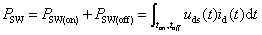(6)

Switching loss PSW of MOSFET Mainly includes opening loss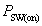and turn off loss. The size of PSW is not only related to drain source voltage of switching tube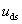and drain current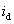, but also related to the opening time ton of the switch tube and the turn off time of toff. the smaller Miller Capacitance MOSFET between grid and drain is, the faster switching speed of MOSFET is, the smaller the switching loss is .

Under the condition of the same power bus voltage US and the same output power, the switching loss of the flyback switching power supply is mainly the turn off loss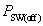. Due to the drain source voltagereduces to close to 0 from the power bus voltage US in the opening process, the drain current rises slowly. Therefore, relative turn off lossand opening lossis very small, almost negligible.

6.2. Comparative Test of the Switching Characteristics of SiC MOSFET and Si MOSFET

6.2.1. Switching Speed of SiC MOSFET and Si MOSFET

The switching speed of SiC MOSFET and Si MOSFET is compared with the test respectively, which shows change of grid source voltage of SiC MOSFET and Si MOSFET in the switching process. Experiment shows that SiC MOSFET is much faster than Si MOSFET. Therefore, the turn off lossof SiC MOSFET will be significantly less than the turn off loss of Si MOSFET.

6.2.2. Comparative Test of the Power Loss of MOSFET

The opening loss, turn off loss, conduction loss and total loss of SiC MOSFET and Si MOSFET in a switching period are calculated by using the test calculation software coming with TPS2024 isolation channel oscilloscope. Because the switch speed of SiC MOFET is significantly faster than Si MOSFET, so there is a significant difference in the turn off loss. The turn off loss of Si MOSFET is 6.26W, and the turn off loss of SiC MOSFET is 61.0W. Due to the limitations in PWM chip driving voltage in the test, the grid drive voltage of MOSFET is 12V, the conduction resistance of SiC MOSFET is about 3 times more than that under the 20V grid voltage. So there are no obvious advantages of SiC MOSFET in conduction loss, both being 2.59W. Due to the switching loss of the flyback switching power supply is mainly the turn off loss, and the opening loss is very small, the opening loss of the two devices is 0 in the test.

If the grid drive voltage of SiC MOSFET is up to 20V, the conduction resistance will be reduced to 1/4 under the 12V grid voltage state.

6.3. Comparative Test of the Efficiency of Switching Power Supply

Compared with the high switching speed of Si MOSFET and SiC MOSFET, the power loss in flyback switching power supply can significantly reduce. In order to verify the effect of the power loss of SiC MOSFET in the switching power supply, we test the switching power supply input power of SiC MOSFET and Si MOSFET respectively .

It shows that the switching power supply input power of SiC MOSFET is 1.28 kW, and the switching power supply input power of Si MOSFET (effective) is 1.33 kW, with a difference of 0.05kW. It is a part that loss of Si MOSFET higher than that of SiC MOSFET, accounting for 3.8% of the total input power. It indicates that even if we directly use SiC MOSFET instead of Si MOSFET, it will also improve the efficiency of nearly 4%, and if the grid drive voltage is up to 20V, the increase of the efficiency will be more obvious.

7. Conclusion

We study the characteristics and parameters of SiC SBD and SiC MOSFET in this paper. The main contents are shown as follows:

1) To describe the research status of the application of SiC power devices, and to point out the problems of the application of SiC power devices.

2) To study the switching characteristics of SiC SBD and SiC MOSFET, and to compare and analyze the switching characteristics of SiC power device and Si power device.

Through the analysis of the power loss characteristics of silicon carbide MPS, the valve loss of high voltage DC silicon carbide thyristor and the power loss of Si MOSFET and SiC MOSFET in the switching power supply. It can be concluded that silicon carbide device has following advantages:

1) We can get the best power loss characteristics by choosing appropriate structure parameters. Therefore, the application of MPS is for high temperature, high power system.

2) If using silicon carbide thyristors to replace silicon thyristors, due to under the condition of the same trigger angle, total power loss is greatly reduced. It can significantly reduce the requirements of cooling equipment, more benefit to the normal operation in the DC system from the aspect of operation, and can save large amounts of electricity per year, bringing economic benefits that can not be ignored.

3) SiC MOSFET is a high voltage switching device with excellent performance. It not only has a good blocking ability and a low turn-on voltage, it also has the extremely fast switching speed, which can be used for high voltage and high frequency switching power converter field.

References

1. Qian Zhaoming, Sheng Kuang. Development and perspective of high power semiconductor device[J]. Converter Technology & Electric Traction, 2010, (1): 1-9.
2. Chen Zhiming, Li Shouzhi. Wide band gap semiconductor power electronic devices and their applications[M]. Beijing: China Machine Press, 2009.
3. Agarwal A K. An overview of SiC power devices[A]. ICPCES 2010[C]. 2010, 1-4.
4. Agarwal A, Callanan R, Das M,et al. Advanced HF SiC MOS devices[A]. Power Electronics and Applications, 13th European Conference 2009[C]. 2009. 1,8-10.
5. Jiang Dung, Burgos R, Wang Fei, et al. Temperature-dependent characteristic of SiC devices: performance evaluation and loss calculation[J]. IEEE Transactions on Power Electronics, 2012, 27(2): 1013-1024.
6. Glaser J S, Nasadoski J J, Losee P A, et al. Direct comparison of silicon and silicon carbide power transistors in high-frequency hard-switched applications[A]. APEC2011[C]. 2011. 1049-1056.
7. Alatise O, Parker-Allotey N A, Mawby P. The dynamic performance of SiC Schottky barrier diodes with parasitic inductances over a wide temperature range[A]. PEMD2012 [C]. 2012, 1-6.
8. Zhao Bin, Qin Haihong, Ma Ceyu, et al. Exploration of switching characteristics of SiC-based power devices[J]. Advanced Technology of Electrical Engineering and Energy, 2014, 33(3): 18-22.
9. http://www.cree.com/.
10. http://www.ixys.com/.
11. Niu Xinjun, Zhang Yuming, Zhang Yinmen, et al. Analysis of Power Dissioation Characteristics of MPS Based on SiC[J]. RESEARCH & PROGRESS OF SSE, 2003, 23(2): 193-198.
12. Zhang Yuming, Study on silicon carbide materials and devices, doctoral dissertation, Xi'an Jiao Tong University, 1998. 4.
13. IEC61803-1999, Determination of power losses in high-voltage direct current converter[S].
14. Cepek M. Loss measurement in high voltage thyristor valves[J]. IEEE Trans on Power Delivery, 1994, 9(3): 1222-1236.
15. Kimbark E W. Direct current transmission: Vol.I[M]. New York: John&Sons, Inc, 1971: 21-25.
16. Uhlmann E. Power transmission by direct current[M]. Heidelberg, New York: Springer-Verlag Berlin, 1995: 37-41.
17. Jin Rui, Lei Linxu, Wen Jialiang, et al. Discussion on Power Loss of HVDC Converter Valves Adopting Silicon Carbide Thyristors[J]. Power System Technology, 2011, 35(3): 8-13.
18. Wen Jialiang, Zha Kunpeng, Gao Chong, et al. Research and development of whole-set operational test UHVDC thyristor valves[J]. Power System Technology, 2010, 34(8): 1-5.
19. Sha Zhanyou, Wang Yanpeng, Ma Hongtao, etc, Optimal design of switching power supply [M]. Beijing: China Electric Power Press, 2009: 260-261.
20. Hosseini Aghdam M G, Thiringer T. Comparison of SiC and Si power semiconductor devices to be used in 2.5 kW DC-DC converter[C]. International Conference on Power Electronics and Drive Systems, PEDS, 2009: 1035-1040.
21. Nathabhat Pankong, Tsuyoshi Funaki, Takashi Hikihara. Characterization of the gate-voltage dependency of input capacitance in a SiC MOSFET[J]. IEICE Electronics Express, 2010, 7(7): 480-486.
22. Cao Hongkui, Chen Zhibo, Meng Linan. Comparative Analysis of SiC MOSFET Power Losses in Switching Power Supply[J]. Journal of Liaoning University of Technology(Natural Science Edition), 2014, 34(2): 82-85.

 Contents 1. 2. 2.1. 2.2. 3. 3.1. 3.2. 4. 4.1. 4.2. 4.3. 5. 5.1. 5.2. 5.3. 5.4. 5.5. 6. 6.1. 6.2. 6.3. 7.
Article ToolsAbstractPDF(600K)